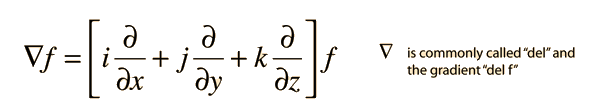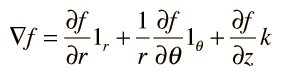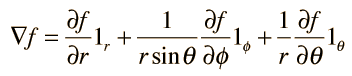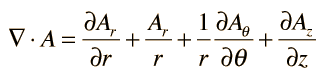The gradient is a vector operation which operates on a scalar function to produce a vector whose magnitude is the maximum rate of change of the function at the point of the gradient and which is pointed in the direction of that maximum rate of change. In rectangular coordinates the gradient of function f(x,y,z) is:If S is a surface of constant value for the function f(x,y,z) then the gradient on the surface defines a vector which is normal to the surface.

 Applications Conduction heat transfer

The divergence of the gradient is called the LaPlacian. It is widely used in physics.

### Gradient in other coordinate systems

Index

Vector calculus

 HyperPhysics*****HyperMath*****Calculus R Nave
Go Back

Compared to the gradient in rectangular coordinates:In cylindrical polar coordinates:and in spherical polar coordinates:Index

Vector calculus

 HyperPhysics*****HyperMath*****Calculus R Nave
Go Back

# Divergence, Various Coordinates

Compared to the divergence in rectangular coordinates:In cylindrical polar coordinates:and in spherical polar coordinates:Index

Vector calculus

 HyperPhysics*****HyperMath*****Calculus R Nave
Go Back